BFOIT - Introduction to Computer Programming

# Java Operators

Operators are mostly special characters, with a couple keywords thrown in, that make something happen.

Connector"the dot" connects classes and objects to members.  The places where you use it in what I cover are:

(1) when you are connecting an object reference variable to a method.  The Extending Existing Classes lesson introduces the dot operator for this use, and

(2) when you are connecting a class name to one of its static fields. An example of this is the dot between "System" and "out" in the statements we use to print stuff to the console window. System is the name of a class included in every Java implementation.  It has an object reference variable that points to a PrintStream object for the console. So, "System.out.println( "text") invokes the println() method of the System.out object.

Object Creationbuilds objects for you. Objects are instantiations of classes and arraysnew is introduced in the Extending Existing Classes lesson.

Assignment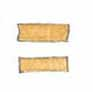is the basic assignment operator. The equals-sign sits between what we call the "lefthand-side" and the "righthand-side" of the assignment expression.

 lefthand-side Op righthand-side = text = "Harry Potter" number = 10 alphabet = 'A'

In all of the Java source we will cover, the righthand-side will be an expression. The lefthand-side will be the name (identifier) of a variable or an array expression which is a name followed by an index expression in square brackets.

The Java compiler will generate bytecode such that when the JVM executes the assignment expression, it

1. computes the lefthand-side (needed to finalize where we are storing the righthand-side value,
2. computes the righthand-side value, and
3. takes this value and stores it into the location we computed in step 1.

Equality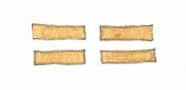sits between two things, compares them and produces true if they are identical or false if they are different.

The Java compiler will generate bytecode such that when the JVM executes the equality expression, it

1. computes the value to the left of the equality operator (the double-equals-sign), then
2. computes the value to the right, and finally
3. compares these two values. If they are the same, the result is true, otherwise false.

So, the value it produces is boolean.

Inequality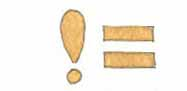sits between two things, compares them and produces true if they are different or false if they are identical.

The Java compiler will generate bytecode such that when the JVM executes the inequality expression, it

1. computes the value to the left of the inequality operator (the bang-equals-sign, aka exclamation-equals-sign, aka NOT-equals-sign), then
2. computes the value to the right, and finally
3. compares these two values. If they are different, the result is true, otherwise false.

So, the value it produces is boolean.

Relational - Less Than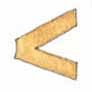sits between two things, compares them and produces true if the thing on the left is less than the thing on the right, otherwise false.

The Java compiler will generate bytecode such that when the JVM executes the less-than expression, it

1. computes the value to the left of the less-than operator ("<"), then
2. computes the value to the right of it, and finally
3. compares these two values. If the first is less than the second, the result is true, otherwise false.

So, the value it produces is boolean.

Relational - Less Than or Equal To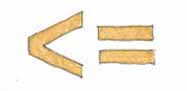sits between two things, compares them and produces true if the thing on the left is less than, or equal to the thing on the right, otherwise false.

The Java compiler will generate bytecode such that when the JVM executes the less-than-or-equal-to expression, it

1. computes the value to the left of the operator ('<='), then
2. computes the value to the right of it, and finally
3. compares these two values. If the first is less than or equal to the second, the result is true, otherwise false.

So, the value it produces is boolean.

Relational - Greater Thansits between two things, compares them and produces true if the thing on the left is greater than the thing on the right, otherwise false.

The Java compiler will generate bytecode such that when the JVM executes the greater-than expression, it

1. computes the value to the left of the greater-than operator ('>'), then
2. computes the value to the right of it, and finally
3. compares these two values. If the first is greater than the second, the result is true, otherwise false.

So, the value it produces is boolean.

Relational - Greater Than or Equal To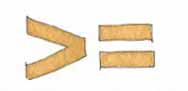sits between two things, compares them and produces true if the thing on the left is greater than, or equal to the thing on the right, otherwise false.

The Java compiler will generate bytecode such that when the JVM executes the greater-than-or-equal-to expression, it

1. computes the value to the left of the operator ('>='), then
2. computes the value to the right of it, and finally
3. compares these two values. If the first is greater than or equal to the second, the result is true, otherwise false.

So, the value it produces is boolean.

Logical - And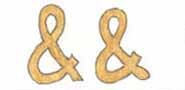sits between two boolean things and replaces the pair of things with a single boolean value.  The value produced is true only if both the left value AND the right value are true, otherwise false.

The Java compiler will generate bytecode such that when the JVM executes the logical-and expression, it

1. computes the value to the left of the logical-and operator ('&&'), then
2. if the value is false, it's done; the expression result is false, otherwise
3. computes the value to the right of it and this value becomes the result.

NOTE that evaluation of the righthand-side is NOT guaranteed.  It may not happen.

So, the value it produces is boolean.

Logical - Orsits between two boolean things and replaces the pair of things with a single boolean value.  The value produced is true if either the left value OR the right value is true, otherwise false if both the right and left are false.

The Java compiler will generate bytecode such that when the JVM executes the logical-and expression, it

1. computes the value to the left of the logical-or operator ('||'), then
2. if the value is true, it's done; the expression result is true, otherwise
3. computes the value to the right of it and this value becomes the result.

NOTE that evaluation of the righthand-side is NOT guaranteed.  It may not happen.

So, the value it produces is boolean.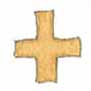sits between two numeric things and replaces the pair of things with a single numeric value.

The Java compiler will generate bytecode such that when the JVM executes the addition expression, it

1. computes the value to the left of the addition operator ('+'),
2. computes the value to the right of the addition operator ('+'), then

So, the value it produces is numeric.

Arithmetic - Subtractionsits between two numeric things and replaces the pair of things with a single numeric value.

The Java compiler will generate bytecode such that when the JVM executes the subtraction expression, it

1. computes the value to the left of the subtraction operator ('-'),
2. computes the value to the right of the subtraction operator ('-'), then
3. subtracts the right-hand value from the left-hand value

So, the value it produces is numeric.

Arithmetic - Multiplicationsits between two numeric things and replaces the pair of things with a single numeric value.

The Java compiler will generate bytecode such that when the JVM executes the multiplication expression, it

1. computes the value to the left of the multiplication operator ('*'),
2. computes the value to the right of the multiplication operator ('*'), then
3. computes the product of these two values

So, the value it produces is numeric.

Arithmetic - Division, Quotient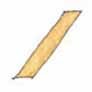sits between two numeric things and replaces the pair of things with a single numeric value.

The Java compiler will generate bytecode such that when the JVM executes the division expression, it

1. computes the value to the left of the division operator ('/'),
2. computes the value to the right of the division operator ('/'), then
3. divides the left-hand value by the right-hand value

So, the value it produces is numeric.

Arithmetic - Division, Remaindersits between two numeric things and replaces the pair of things with a single numeric value.

The Java compiler will generate bytecode such that when the JVM executes the division expression, it

1. computes the value to the left of the division operator ('%'),
2. computes the value to the right of the division operator ('%'), then
3. divides the left-hand value by the right-hand value
4. the remainder produced by this division is the final value

So, the value it produces is numeric.

Increment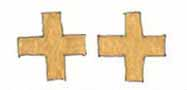when appended to a variable identifier, the variable is incremented by 1.  Think of it as an abbreviation for the assignment statement:

variableIdentifier = variableIdentifer + 1;

Decrementwhen appended to a variable identifier, the variable is decremented by 1.  Think of it as an abbreviation for the assignment statement:

variableIdentifier = variableIdentifer - 1;